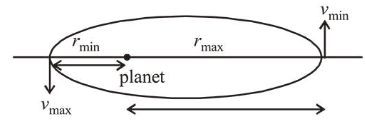# A satellite is in an elliptical orbit around a planet P.

Question:

A satellite is in an elliptical orbit around a planet $P$. It is observed that the velocity of the satellite when it is farthest from the planet is 6 times less than that when it is closest to the planet. The ratio of distances between the satellite and the planet at closest and farthest points is :

1. (1) $1: 6$

2. (2) $1: 3$

3. (3) $1: 2$

4. (4) $3: 4$

Correct Option: 1

Solution:

(1) By angular momentum conservation

$r_{\min } v_{\max }=m r_{\max } v_{\min }$Given, $v_{\min }=\frac{v_{\max }}{6}$

$\therefore \frac{r_{\min }}{r_{\max }}=\frac{v_{\min }}{v_{\max }}=\frac{1}{6}$